### Home > AC > Chapter 9 > Lesson 9.1.1 > Problem9-10

9-10.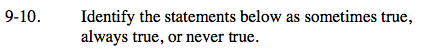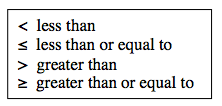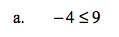−4 is always less than or equal to 9 on the number line.
Therefore, the statement is always true.

Always true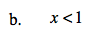The answer is true depending on what x equals.
If x is 1 or higher, the statement is not true.
If it is any other number, the statement is true. Therefore, it is sometimes true.

Sometimes true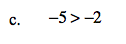Follow the reasoning in part (a).

Never true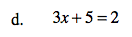3x + 5 = 2
−5 −5
3x = −3

If x is equal to −1, then the statement is true.
Any other time the statement is false.
Therefore, the statement is sometimes true.

Sometimes true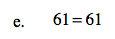Always true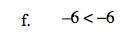Follow the reasoning in part (a).